# Introduction

Many employees often complain about the process of promoting staff in their respective organization. Promotion is the advancement of an employee’s rank or position in an organizational hierarchy system, usually an employee’s reward for outstanding performance. In this article, we fitted four machine learning algorithms and selected the best algorithm to explain relevant features to Staff promotion using the DSN data set. The dataset can be downloaded via this link. Let’s start by loading the packages and data set into R.

library(forcats)
library(recipes)
library(ggplot2)
library(tidyquant)
library(caret)
library(dplyr)
library(lime)
library(arules)
library(xgboost)
library(pROC)
library(gridExtra)
library(corrr)
library(lime)
dt_new<-read.csv("HRdata.csv", header = T)
str(dt_new)
## 'data.frame':    7662 obs. of  19 variables:
##  $EmployeeNo : Factor w/ 7662 levels "YAK/S/00004",..: 71 1299 2653 2955 5887 5356 6170 5558 1923 5391 ... ##$ Division                           : Factor w/ 9 levels "Business Finance Operations",..: 3 9 4 5 2 2 4 2 2 3 ...
##  $Qualification : Factor w/ 4 levels "","First Degree or HND",..: 2 3 2 3 2 3 1 3 2 2 ... ##$ Gender                             : Factor w/ 2 levels "Female","Male": 2 2 2 1 2 2 2 2 1 1 ...
##  $Channel_of_Recruitment : Factor w/ 3 levels "Agency and others",..: 1 1 1 2 2 2 1 2 2 2 ... ##$ Trainings_Attended                 : int  2 2 2 2 4 2 2 2 2 2 ...
##  $Year_of_birth : int 1991 1986 1982 1987 1980 1980 1993 1985 1990 1994 ... ##$ Last_performance_score             : num  12.5 5 7.5 10 7.5 10 10 2.5 10 0 ...
##  $Year_of_recruitment : int 2015 2010 2016 2012 2013 2016 2017 2009 2013 2018 ... ##$ Targets_met                        : int  0 0 0 1 0 0 1 0 1 0 ...
##  $Previous_Award : int 0 0 0 0 0 0 0 0 0 0 ... ##$ Training_score_average             : int  47 62 78 71 37 43 77 42 44 53 ...
##  $State_Of_Origin : Factor w/ 37 levels "ABIA","ADAMAWA",..: 14 25 31 23 19 31 15 12 25 25 ... ##$ Foreign_schooled                   : Factor w/ 2 levels "No","Yes": 2 2 2 2 2 2 2 2 2 1 ...
##  $Marital_Status : Factor w/ 3 levels "Married","Not_Sure",..: 1 1 1 1 1 1 1 1 1 1 ... ##$ Past_Disciplinary_Action           : Factor w/ 2 levels "No","Yes": 1 1 1 1 1 1 1 1 1 1 ...
##  $Previous_IntraDepartmental_Movement: Factor w/ 2 levels "No","Yes": 1 1 1 1 1 1 1 1 1 1 ... ##$ No_of_previous_employers           : Factor w/ 7 levels "0","1","2","3",..: 1 1 2 1 1 1 2 2 3 2 ...
##  $Promoted_or_Not : int 0 0 0 0 0 0 0 0 0 0 ... The data contains 7662 observations and 19 variables. The target variable “Promoted_or_Not” is seen as an integer, hence we need to convert it to factor and then check for missing values. dt_new$Promoted_or_Not<-as.factor(dt_new$Promoted_or_Not) sapply(dt_new, function(x) sum(is.na(x))) ## EmployeeNo Division ## 0 0 ## Qualification Gender ## 0 0 ## Channel_of_Recruitment Trainings_Attended ## 0 0 ## Year_of_birth Last_performance_score ## 0 0 ## Year_of_recruitment Targets_met ## 0 0 ## Previous_Award Training_score_average ## 0 0 ## State_Of_Origin Foreign_schooled ## 0 0 ## Marital_Status Past_Disciplinary_Action ## 0 0 ## Previous_IntraDepartmental_Movement No_of_previous_employers ## 0 0 ## Promoted_or_Not ## 0 Using the sapply function to check for missing values, we observed that there are no missing values in our data set. Looking at our data, we’ve got some wranglings to do. First of all, let’s remove the variable “EmployeeNo”, it won’t be necessary for our analysis. Also, we will be adding new variables. The first variable to be added is “Age” which will be obtained from “Year_of_birth, we will also be adding another variable called”Year_exp" which measures years of experience, it will be derived from “Year_of_recruitment”. we will like to know if employees’ Age and years of experience play any significant role in the promotion process. Finally, we will be grouping the variable “State_of_Origin” into two categories, north and south. We want to know if the region they come from plays a significant role in the promotion process. So Let’s add the variables dt_new$EmployeeNo<-NULL
dt_new<-dt_new%>%mutate(Age= 2019 - Year_of_birth)
dt_new<-dt_new%>%mutate(Exp_Age= 2019 - Year_of_recruitment)
dt_new<-dt_new%>% mutate(State_group = case_when(State_Of_Origin %in% c("ANAMBRA","AKWA IBOM","ENUGU","OYO","LAGOS","ONDO","EDO","RIVERS" ,"EKITI","IMO", "CROSS RIVER", "OGUN", "DELTA","OSUN","ABIA","BAYELSA" ,"EBONYI")~1, State_Of_Origin %in% c("KATSINA","NIGER","KWARA","BAUCHI","TARABA","KADUNA","PLATEAU","BORNO","KANO","FCT","GOMBE","BENUE","ZAMFARA","KEBBI","ADAMAWA","NASSARAWA","SOKOTO","KOGI","JIGAWA","YOBE")~2))
dt_new$State_group <- factor(dt_new$State_group , labels=c("South","North"))

# Exploratory Data Analysis

Let’s start by discretizing the variables “Age” and “Exp_Age”. We will be grouping Age into three categories and Exp_Age into four categories. Why do we have to discretize these variables? From experience, the impact of continuous variables such as age and years of experience are better understood when discretized into meaningful groups.

dt_new<- dt_new%>% mutate(agegroup = case_when(Age >= 18  & Age <= 35 ~ '1', Age >= 36  & Age <= 52 ~ '2', Age >= 53  & Age <= 69 ~ '3'))
dt_new$agegroup<- factor(dt_new$agegroup, labels=c("Young", "Middle-Aged","Old"))
dt_new<- dt_new%>% mutate(Exp_Agegroup = case_when(Exp_Age >= 1  & Exp_Age <= 9 ~ '1', Exp_Age >= 10  & Exp_Age<= 18 ~ '2',Exp_Age >= 19  & Exp_Age <= 27 ~ '3',Exp_Age >= 28  & Exp_Age <= 37 ~ '4'))
dt_new$Exp_Agegroup<- factor(dt_new$Exp_Agegroup, labels=c("Less than 10yrs", "Between 10-18yrs","Between 19-27yrs", "Between 28-37yrs"))

The variables “Age” and “Exp_Age” were derived from “Year_of_birth” and " Year_of_recruitment" respectively. Hence we will be dropping “Year_of_birth” and " Year_of_recruitment“. Also, since we have discretized”Age" and “Exp_Age”, we will also be dropping them from our data set and work with the discretized version. Remember that we have also categorized the variable “State_Of_Origin” into two groups, North and South. Hence we will be dropping “State_Of_Origin” and work with the transformed variable “State_group”. Let’s do the dropping.

dt_new$State_Of_Origin<-NULL dt_new$Year_of_birth<-NULL
dt_new$Year_of_recruitment<-NULL dt_new$Age<-NULL
dt_new$Exp_Age<-NULL Let’s Examine the distribution of our categorical variables p1 <- ggplot(dt_new, aes(x=Exp_Agegroup)) + ggtitle("Years of Experience") + xlab("Experience Group") + geom_bar(aes(y = 100*(..count..)/sum(..count..)), width = 0.5) + ylab("Percentage") + coord_flip() + theme_minimal() p2 <- ggplot(dt_new, aes(x=agegroup)) + ggtitle("Age") + xlab("Age Group") + geom_bar(aes(y = 100*(..count..)/sum(..count..)), width = 0.5) + ylab("Percentage") + coord_flip() + theme_minimal() p3 <- ggplot(dt_new, aes(x=State_group)) + ggtitle("Region") + xlab("Region") + geom_bar(aes(y = 100*(..count..)/sum(..count..)), width = 0.5) + ylab("Percentage") + coord_flip() + theme_minimal() p4 <- ggplot(dt_new, aes(x=Foreign_schooled )) + ggtitle("Foreign Schooled") + xlab("Foreign Schooled") + geom_bar(aes(y = 100*(..count..)/sum(..count..)), width = 0.5) + ylab("Percentage") + coord_flip() + theme_minimal() p5 <- ggplot(dt_new, aes(x=Qualification )) + ggtitle("Qualification ") + xlab("Qualification ") + geom_bar(aes(y = 100*(..count..)/sum(..count..)), width = 0.5) + ylab("Percentage") + coord_flip() + theme_minimal() grid.arrange(p1, p2, p3, p4, p5,ncol=3)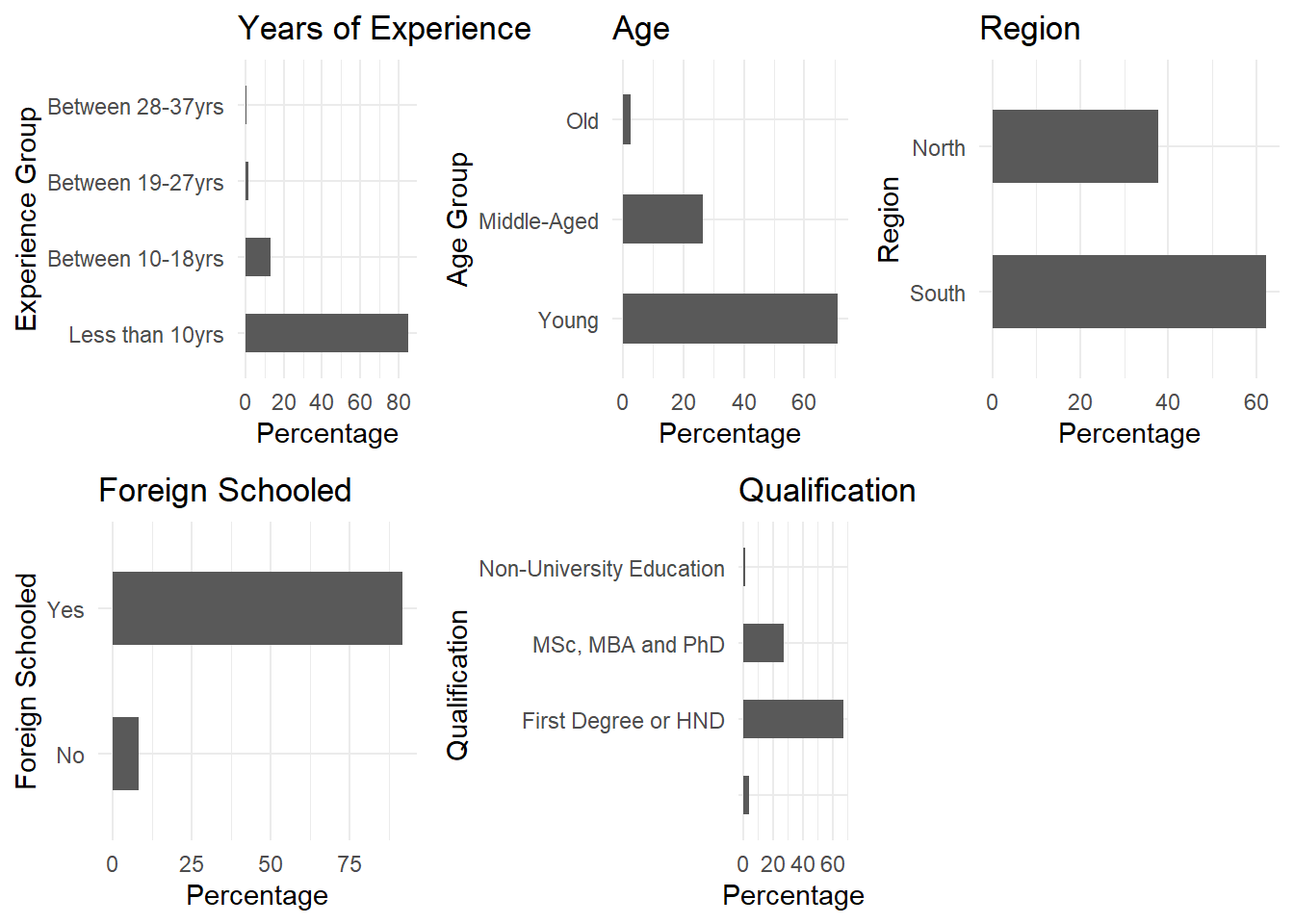p6 <- ggplot(dt_new, aes(x=Marital_Status)) + ggtitle("Marital Status") + xlab("Marital Status") + geom_bar(aes(y = 100*(..count..)/sum(..count..)), width = 0.5) + ylab("Percentage") + coord_flip() + theme_minimal() p7 <- ggplot(dt_new, aes(x=Past_Disciplinary_Action )) + ggtitle("Past Disciplinary Action") + xlab("Past Disciplinary Action") + geom_bar(aes(y = 100*(..count..)/sum(..count..)), width = 0.5) + ylab("Percentage") + coord_flip() + theme_minimal() p8 <- ggplot(dt_new, aes(x= Previous_IntraDepartmental_Movement)) + ggtitle(" Previous IntraDepartmental Movement") + xlab(" Previous IntraDepartmental Movement") + geom_bar(aes(y = 100*(..count..)/sum(..count..)), width = 0.5) + ylab("Percentage") + coord_flip() + theme_minimal() p9<- ggplot(dt_new, aes(x=Gender)) + ggtitle("Gender") + xlab(" Gender") + geom_bar(aes(y = 100*(..count..)/sum(..count..)), width = 0.5) + ylab("Percentage") + coord_flip() + theme_minimal() p10<- ggplot(dt_new, aes(x=Channel_of_Recruitment)) + ggtitle("Channel of Recruitment") + xlab("Channel of Recruitment") + geom_bar(aes(y = 100*(..count..)/sum(..count..)), width = 0.5) + ylab("Percentage") + coord_flip() + theme_minimal() p11<- ggplot(dt_new, aes(x=Division )) + ggtitle("Division") + xlab("Division ") + geom_bar(aes(y = 100*(..count..)/sum(..count..)), width = 0.5) + ylab("Percentage") + coord_flip() + theme_minimal() grid.arrange(p6,p7,p8,p9,p10, p11, ncol=2)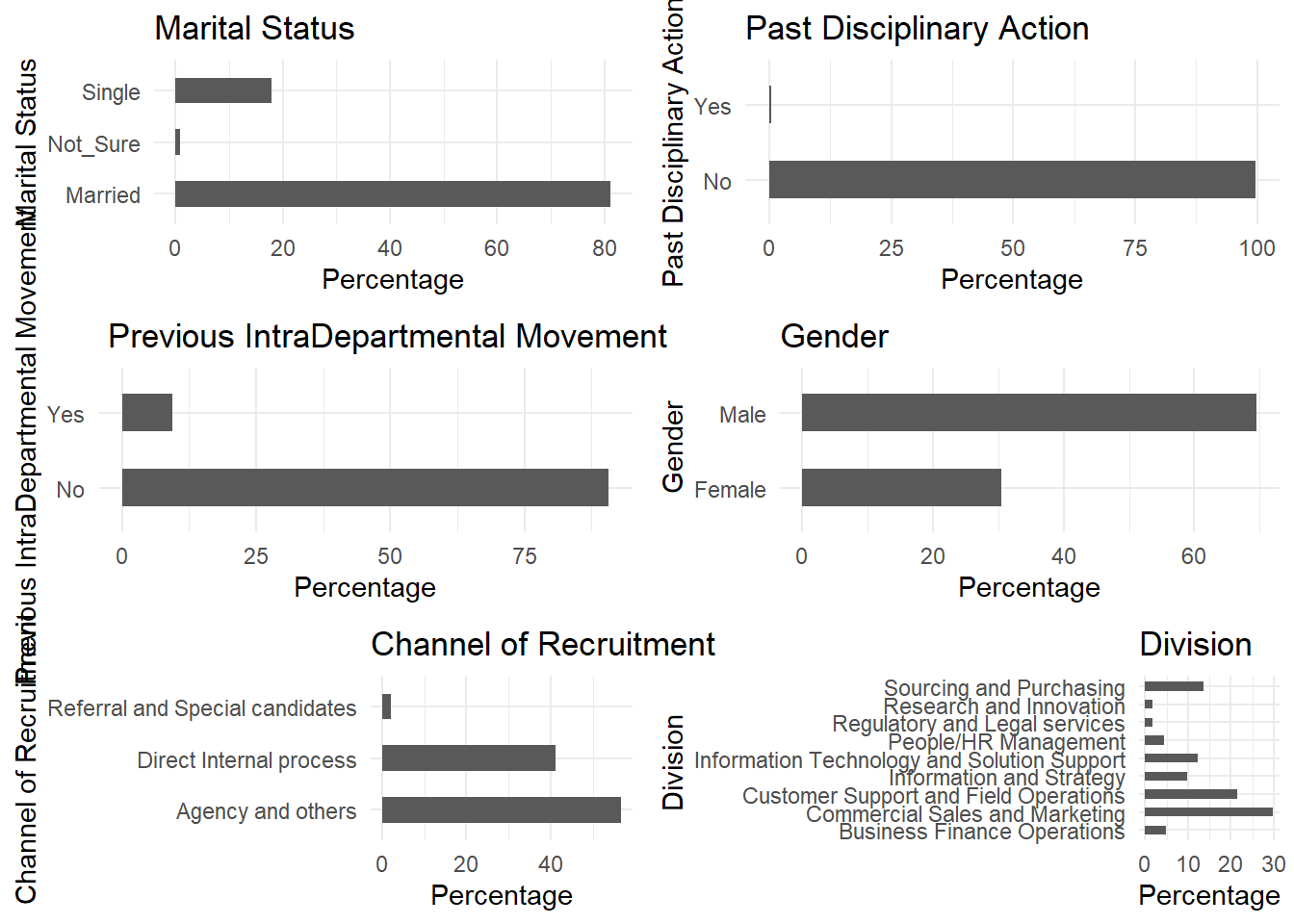We have five features that are multi-category: Channel_of_Recruitment , Qualification, Division, Experience Level, Age Group and Marital Status , many of these categorical variables appear not to have a reasonably broad distribution. We will perform one-hot encoding on the categorical variables.One-hot encoding is the process of converting categorical data into dummy variables which has columns of only zeros and ones. Let’s examine the distribution of the numerical variables p12 <- ggplot(dt_new, aes(x= Trainings_Attended)) + ggtitle("Trainings Attended") + xlab("Trainings Attended") + geom_histogram() + theme_minimal() p13 <- ggplot(dt_new, aes(x=Training_score_average)) + ggtitle("Training score average") + xlab("Training score average") + geom_histogram() + theme_minimal() p14 <- ggplot(dt_new, aes(x=Last_performance_score)) + ggtitle("Last Performance Score") + xlab("Last Performance Score") + geom_histogram() + theme_minimal() grid.arrange(p12,p13, p14)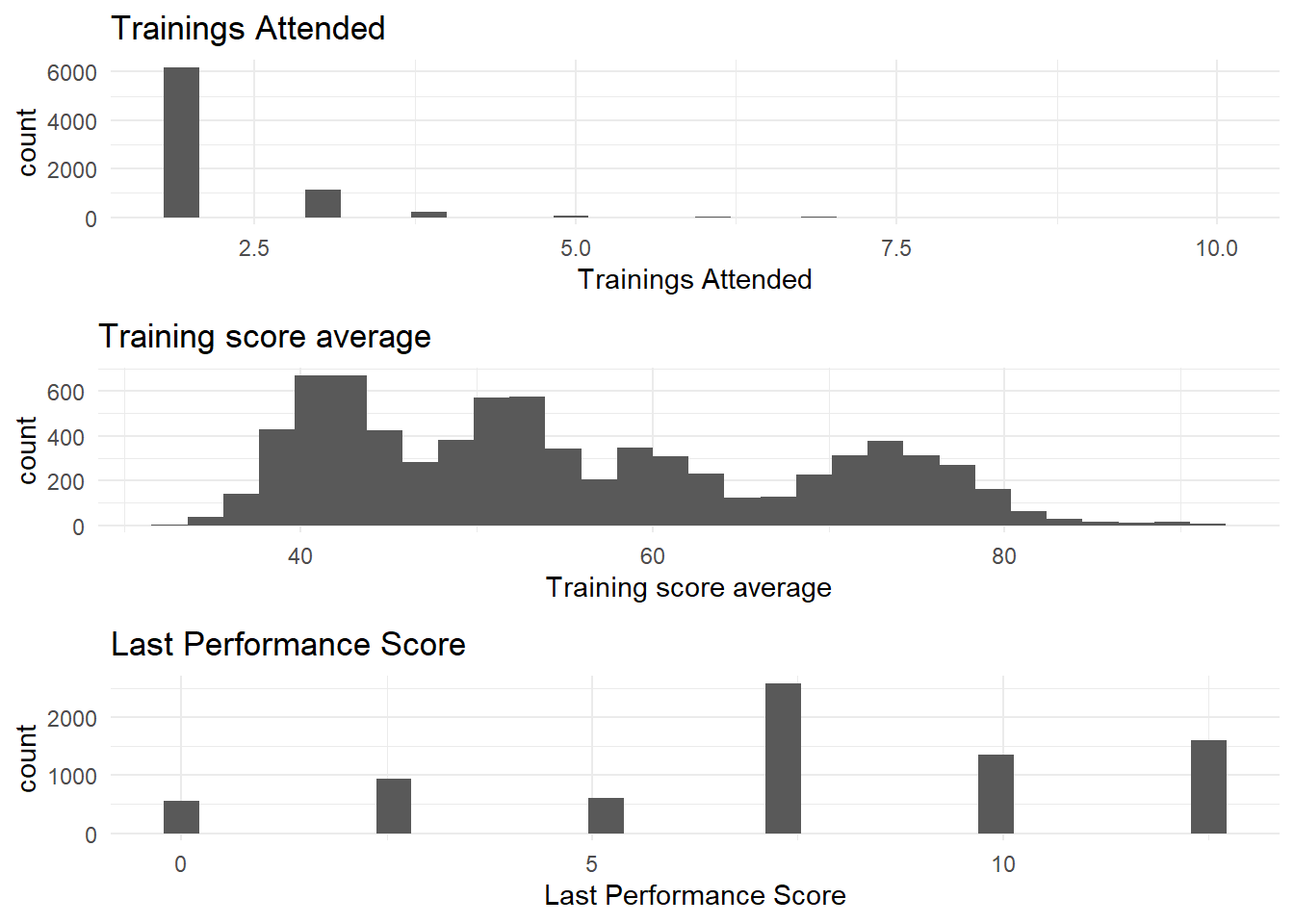The variable Trainings_Attended is skewed to the left, we will apply a log transformation to even out the data. Training_score_average and Last_performance_score are widely spread , hence no need for log transformation. To perform the one-hot encoding and log transformation, we will be using the recipe package. The recipe() function implements the preprocessing steps while the bake() function processes the data by following the steps in the recipe() function. To start the preprocessing, let’s split our data set into training and testing sets. set.seed(1005) split <- sample(seq_len(nrow(dt_new)), size = floor(0.70 * nrow(dt_new))) train <- dt_new[split, ] test <- dt_new[-split, ] dim(train); dim(test) ##  5363 18 ##  2299 18 We set seed for the sake of reproducibility of results. # The Preprocessing data_recipe<- recipe(Promoted_or_Not ~ ., data = train) %>% step_log(Trainings_Attended) %>% step_dummy(all_nominal(), -all_outcomes()) %>% step_center(all_predictors(), -all_outcomes()) %>% step_scale(all_predictors(), -all_outcomes()) %>% prep(data = train) Step_log() to log transform “Trainings_Attended”, step_dummy() to convert categorical variables to dummy variables. step_center() to mean-center the data and step_scale() to scale the data. The centering and scaling were done for the sake of improving numerical stability. # Baking the recipe object train_b <- bake(data_recipe, new_data = train) test_b <- bake(data_recipe, new_data = test) glimpse(train_b) ## Observations: 5,363 ## Variables: 37 ##$ Trainings_Attended                                     <dbl> -0.4516326, ...
## $Last_performance_score <dbl> 0.62877866, ... ##$ Targets_met                                            <dbl> -0.7286113, ...
## $Previous_Award <dbl> -0.1519161, ... ##$ Training_score_average                                 <dbl> -0.61525314,...
## $Promoted_or_Not <fct> 0, 0, 0, 0, ... ##$ Division_Commercial.Sales.and.Marketing                <dbl> 1.5205615, 1...
## $Division_Customer.Support.and.Field.Operations <dbl> -0.5356898, ... ##$ Division_Information.and.Strategy                      <dbl> -0.3244962, ...
## $Division_Information.Technology.and.Solution.Support <dbl> -0.370035, -... ##$ Division_People.HR.Management                          <dbl> -0.2130977, ...
## $Division_Regulatory.and.Legal.services <dbl> -0.1399218, ... ##$ Division_Research.and.Innovation                       <dbl> -0.1276483, ...
## $Division_Sourcing.and.Purchasing <dbl> -0.3896393, ... ##$ Qualification_First.Degree.or.HND                      <dbl> -1.4228279, ...
## $Qualification_MSc..MBA.and.PhD <dbl> 1.6241635, -... ##$ Qualification_Non.University.Education                 <dbl> -0.1174608, ...
## $Gender_Male <dbl> 0.6671432, -... ##$ Channel_of_Recruitment_Direct.Internal.process         <dbl> 1.1870095, -...
## $Channel_of_Recruitment_Referral.and.Special.candidates <dbl> -0.148017, -... ##$ Foreign_schooled_Yes                                   <dbl> 0.2996705, 0...
## $Marital_Status_Not_Sure <dbl> -0.0960167, ... ##$ Marital_Status_Single                                  <dbl> -0.472515, -...
## $Past_Disciplinary_Action_Yes <dbl> -0.05638577,... ##$ Previous_IntraDepartmental_Movement_Yes                <dbl> -0.3283426, ...
## $No_of_previous_employers_X1 <dbl> 1.0117226, -... ##$ No_of_previous_employers_X2                            <dbl> -0.2266118, ...
## $No_of_previous_employers_X3 <dbl> -0.2145279, ... ##$ No_of_previous_employers_X4                            <dbl> -0.1967993, ...
## $No_of_previous_employers_X5 <dbl> -0.1499781, ... ##$ No_of_previous_employers_More.than.5                   <dbl> -0.100844, -...
## $State_group_North <dbl> -0.7784963, ... ##$ agegroup_Middle.Aged                                   <dbl> 1.6686034, -...
## $agegroup_Old <dbl> -0.1649023, ... ##$ Exp_Agegroup_Between.10.18yrs                          <dbl> 2.6152185, -...
## $Exp_Agegroup_Between.19.27yrs <dbl> -0.1253665, ... ##$ Exp_Agegroup_Between.28.37yrs                          <dbl> -0.04928949,...

Great, let’s start the modeling.

# Cross Validation

For the resampling, we will be using the repeated cross-validation, we want it to be repeated once and the number of resampling iterations to be 3.

cv.ctrl <- trainControl(method = "repeatedcv", repeats = 1,number = 3)

# Fitting the Logistic Regression Model

logis<- train(form=Promoted_or_Not~., data=train_b,method="glm", family="binomial",trControl=cv.ctrl)

The Method = “glm” indicates a generalized linear model and family=“binomial” specify the additive logistic regression.

Assessing the Accuracy of our Logistic regression model using the test data set.

predlog<-predict(logis,test_b,type="raw")
confusionMatrix(predlog, test_b$Promoted_or_Not) ## Confusion Matrix and Statistics ## ## Reference ## Prediction 0 1 ## 0 2093 138 ## 1 16 52 ## ## Accuracy : 0.933 ## 95% CI : (0.922, 0.9429) ## No Information Rate : 0.9174 ## P-Value [Acc > NIR] : 0.002881 ## ## Kappa : 0.3759 ## ## Mcnemar's Test P-Value : < 2.2e-16 ## ## Sensitivity : 0.9924 ## Specificity : 0.2737 ## Pos Pred Value : 0.9381 ## Neg Pred Value : 0.7647 ## Prevalence : 0.9174 ## Detection Rate : 0.9104 ## Detection Prevalence : 0.9704 ## Balanced Accuracy : 0.6330 ## ## 'Positive' Class : 0 ##  We’ve got an accuracy of 93.3% from the logistic regression model. Reasonably good right? Let’s examine the accuracy of other models. # Fitting Support Vector Machine svm<- train(form=Promoted_or_Not~., data=train_b, method="svmLinear", trControl=cv.ctrl ) The Method = “svmLinear” indicates a SVM Linear classifier. The trControl is the resampling method we set earlier on. Let’s examine the accuracy of our SVM model. predsvm<-predict(svm,test_b,type="raw") confusionMatrix(predsvm, test_b$Promoted_or_Not)
## Confusion Matrix and Statistics
##
##           Reference
## Prediction    0    1
##          0 2109  155
##          1    0   35
##
##                Accuracy : 0.9326
##                  95% CI : (0.9215, 0.9425)
##     No Information Rate : 0.9174
##     P-Value [Acc > NIR] : 0.003676
##
##                   Kappa : 0.2929
##
##  Mcnemar's Test P-Value : < 2.2e-16
##
##             Sensitivity : 1.0000
##             Specificity : 0.1842
##          Pos Pred Value : 0.9315
##          Neg Pred Value : 1.0000
##              Prevalence : 0.9174
##          Detection Rate : 0.9174
##    Detection Prevalence : 0.9848
##       Balanced Accuracy : 0.5921
##
##        'Positive' Class : 0
## 

For the SVM model, We’ve got an accuracy of 93.26% , a bit lower than the logistic regression model. Let’s examine the performance of the remaining two models, Random forest and Xgboost.

# Fitting Random Forest

mtry <- sqrt(ncol(train_b))
tunegrid <- expand.grid(.mtry=mtry)
rf<- train(form=Promoted_or_Not~., data=train_b,method="rf", metric="Accuracy",tuneGrid=tunegrid, trControl=cv.ctrl)

The mtry argument specifies the number of variables to be randomly selected from the set of predictors available when forming each split in a tree for the random forest. In our own case, we want it to be the square root of the number of variables in our training data set. The method=“rf” specifies that the random forest model should be fitted.

Let’s also examine the performance of the random forest model using the test data set.

predrf<-predict(rf,test_b,type="raw")
confusionMatrix(predlog, test_b$Promoted_or_Not) ## Confusion Matrix and Statistics ## ## Reference ## Prediction 0 1 ## 0 2093 138 ## 1 16 52 ## ## Accuracy : 0.933 ## 95% CI : (0.922, 0.9429) ## No Information Rate : 0.9174 ## P-Value [Acc > NIR] : 0.002881 ## ## Kappa : 0.3759 ## ## Mcnemar's Test P-Value : < 2.2e-16 ## ## Sensitivity : 0.9924 ## Specificity : 0.2737 ## Pos Pred Value : 0.9381 ## Neg Pred Value : 0.7647 ## Prevalence : 0.9174 ## Detection Rate : 0.9104 ## Detection Prevalence : 0.9704 ## Balanced Accuracy : 0.6330 ## ## 'Positive' Class : 0 ##  The performance of the random forest on the test data set is the same as that of logistic regression. Let’s fit our final model, Xgboost. # Fitting Xgboost Let’s start by defining some of the parameters in the Xgboost model. nthread: Activates parallel computation. nround: The number of trees to grow, we want it to be 100. eta: lies between 0.01 - 0.3, controls learning rate, it indicates the rate at which the model is learning from data. We want to leave it at the default value of 0.3. max_depth: It controls the depth of the tree, we want it at the default value of 6. Min_child_weight: it blocks the potential feature interactions to prevent overfitting. Let’s leave it at the default value of 1. subsample: It controls fraction of observations to be randomly sampled for each tree. Let’s leave it at the default value of 1. colsample_bytree: It controls the number of features (variables) supplied to a tree. Let’s use the default of 1. xgb.grid <- expand.grid(nrounds = 100,eta=0.3, gamma=0, max_depth=3, min_child_weight=1, subsample=1, colsample_bytree=1) xgb_model <-train(Promoted_or_Not ~ .,data = train_b, method="xgbTree",trControl=cv.ctrl, tuneGrid=xgb.grid,nthread =4) Let’s examine the performance of the XGBoost model predxgb<-predict(xgb_model,test_b,type="raw") confusionMatrix(predxgb, test_b$Promoted_or_Not)
## Confusion Matrix and Statistics
##
##           Reference
## Prediction    0    1
##          0 2104  135
##          1    5   55
##
##                Accuracy : 0.9391
##                  95% CI : (0.9285, 0.9485)
##     No Information Rate : 0.9174
##     P-Value [Acc > NIR] : 4.696e-05
##
##                   Kappa : 0.4169
##
##  Mcnemar's Test P-Value : < 2.2e-16
##
##             Sensitivity : 0.9976
##             Specificity : 0.2895
##          Pos Pred Value : 0.9397
##          Neg Pred Value : 0.9167
##              Prevalence : 0.9174
##          Detection Rate : 0.9152
##    Detection Prevalence : 0.9739
##       Balanced Accuracy : 0.6436
##
##        'Positive' Class : 0
## 

Based on the Accuracy rate, Xgboost performs better than the previous three models fitted.

# ROC Curve for the Fitted Models.

## ROC Curve for SVM
response1 <- predictor1 <- c()
response1 <- c(response1, test_b$Promoted_or_Not) predictor1<- c(predictor1,predsvm) roc1 <- plot.roc(response1, predictor1, main="ROC Curve for the Fitted Models",ylab="True Positive Rate",xlab="False Positive Rate", percent=TRUE, col="green") ## ROC Curve for Random Forest response2 <- predictor2 <- c() response2 <- c(response2, test_b$Promoted_or_Not)
predictor2<- c(predictor2, predrf)
par(new=T)
roc2<- plot.roc(response2, predictor2, ylab="True Positive Rate",xlab="False Positive Rate", percent=TRUE, col="red")

## ROC Curve for Logistic Regression
response3 <- predictor3 <- c()
response3 <- c(response3, test_b$Promoted_or_Not) predictor3 <- c(predictor3, predlog) par(new=T) roc3<- plot.roc(response3, predictor3, ylab="True Positive Rate",xlab="False Positive Rate", percent=TRUE, col="magenta") ## ROC Curve for Xgboost response4 <- predictor4 <- c() response4 <- c(response4, test_b$Promoted_or_Not)
predictor4 <- c(predictor4, predxgb)
par(new=T)
roc4<- plot.roc(response4, predictor4,ylab="True Positive Rate",xlab="False Positive Rate", percent=TRUE, col="blue")
legend("bottomright", legend = c("XGBoost", "Random Forest", "SVM", "LogR"), col = c("blue", "red", "green", "magenta"),lwd = 2)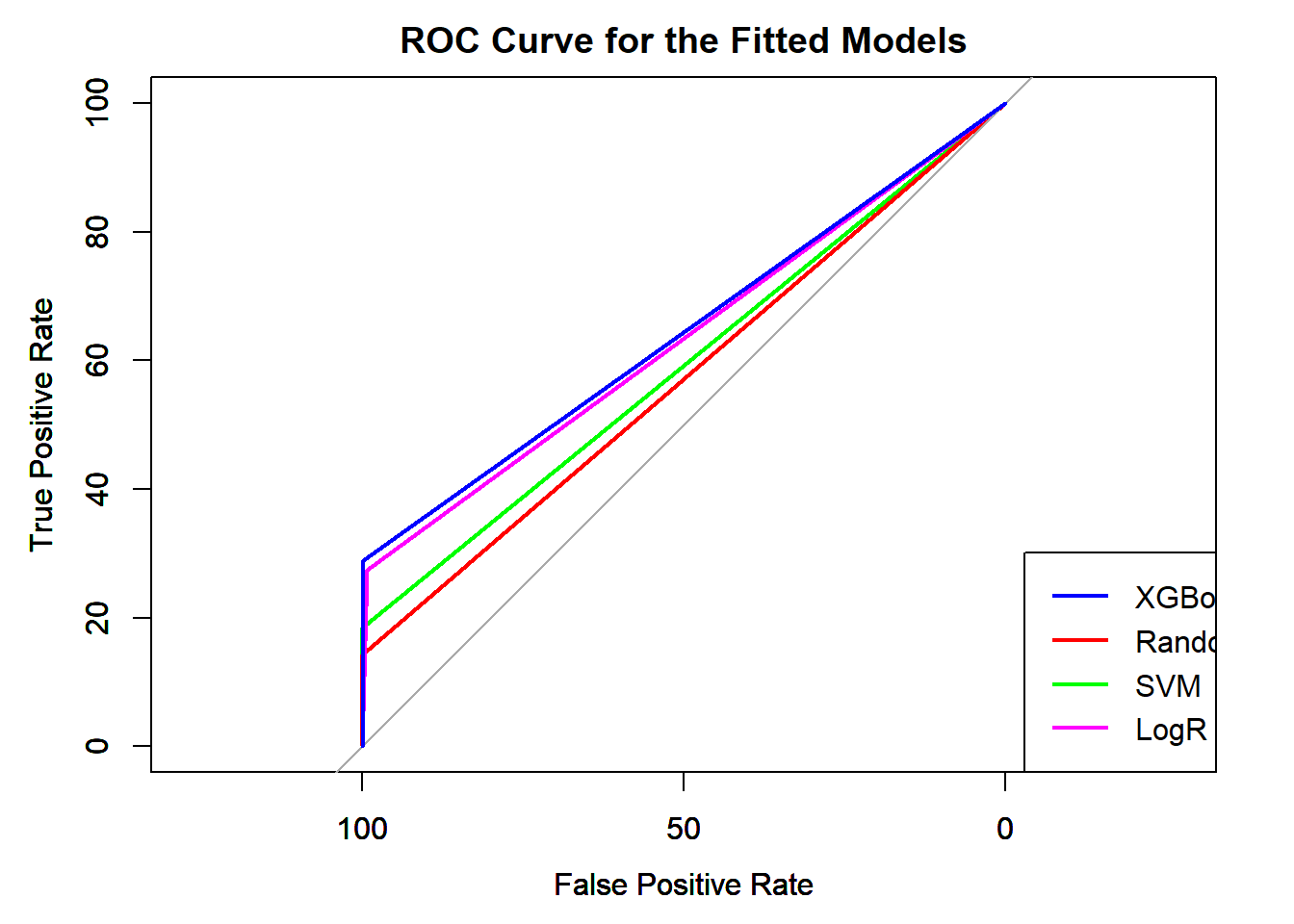The Xgboost has the highest accuracy as seen from the output above, also from the ROC curve, the Xgboost model has the largest area under the curve. Going forward, the Xgboost algorithm is recommended. Let’s examine the Xgboost model and the most influential features locally using LIME.

# Model Explanation using the Lime Package

explainer <- lime::lime(
x              =train_b,
model          = xgb_model,
bin_continuous = F,
)

we create an explainer using the lime() function, which only takes the model we intend to explain which is the Xgboost model and the train data set. We set the bin_continuous = FALSE.

Let’s examine factors that were important to being promoted by selecting five cases in our test data set.

explanation <- lime::explain(
test_b[1:5, ],
explainer    = explainer,
n_features   = 6,
feature_select = "highest_weights",
labels = "1"
)

The explain() function helps in explaining the explainer we set above. We set feature_select = “highest_weights” because we are interested in features with the highest absolute weight. We set n_features = 6 because we want to see the six most important features in the XGBoost model. Finally, we set the labels = “1” because we are interested in cases where the staff was promoted.

plot_features(explanation) +
labs(title = "Feature Importance for the Xgboost Model",
subtitle = " Selected four cases")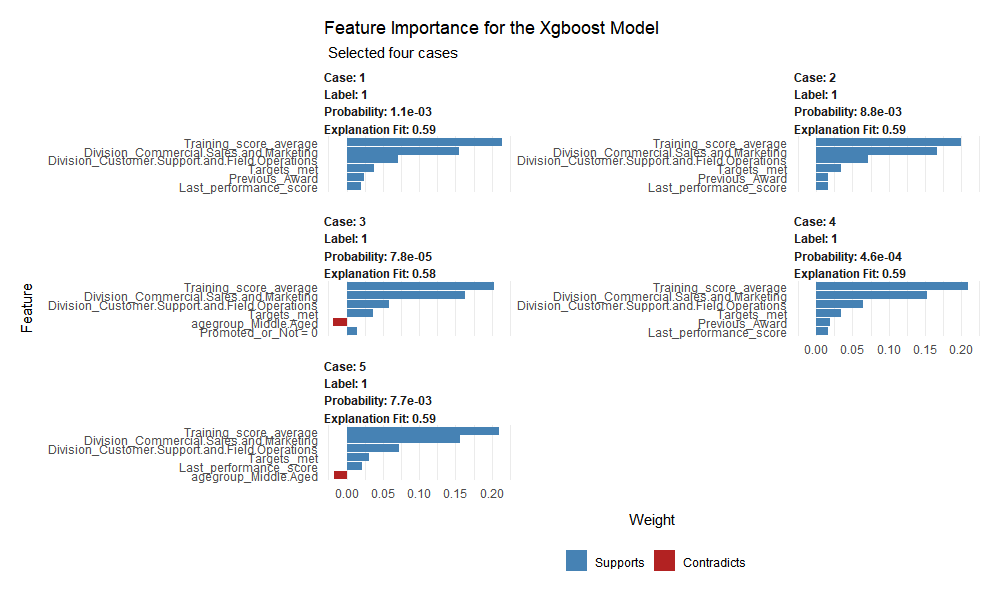For case 1, the six most important features toward being promoted are Training Score Average, Division Commercial Sales and Marketing, Division_Suport and Field Operations, Target_met, Previous_Award, and Last_performance_score. For cases 3 and 4, Age_group(Middle_aged) had a negative impact on their promotion process. The LIME only provides local interpretation which means that we are only interpreting the XGBoost model on a case by case basis. Let’s examine the global interpretation of the Xgboost model, understanding the features that are important on a global perspective using the Corrr package.

train_b$Promoted_or_Not<-as.numeric(train_b$Promoted_or_Not)
global_perspective <- train_b %>%
correlate() %>%
focus(Promoted_or_Not) %>%
rename(Variable=rowname) %>%
arrange(abs(Promoted_or_Not)) %>%
mutate(feature = as.factor(Variable))
global_perspective
## # A tibble: 36 x 3
##    Variable                        Promoted_or_Not feature
##    <chr>                                     <dbl> <fct>
##  1 No_of_previous_employers_X3            0.000113 No_of_previous_employers_X3
##  2 Division_Customer.Support.and.~       -0.00184  Division_Customer.Support.an~
##  3 No_of_previous_employers_X5           -0.00226  No_of_previous_employers_X5
##  4 agegroup_Old                           0.00251  agegroup_Old
##  5 Marital_Status_Single                  0.00412  Marital_Status_Single
##  6 Division_Sourcing.and.Purchasi~        0.00442  Division_Sourcing.and.Purcha~
##  7 Division_Research.and.Innovati~       -0.00498  Division_Research.and.Innova~
##  8 Foreign_schooled_Yes                   0.00629  Foreign_schooled_Yes
##  9 Qualification_First.Degree.or.~       -0.00658  Qualification_First.Degree.o~
## 10 Channel_of_Recruitment_Direct.~       -0.00681  Channel_of_Recruitment_Direc~
## # ... with 26 more rows

Let’s visualize this correlation to enable us identify variables that are relevant to Staff Promotion.

global_perspective %>%
ggplot(aes(x = Promoted_or_Not, y = fct_reorder(Variable, desc(Promoted_or_Not)))) +
geom_point() +  geom_segment(aes(xend = 0, yend = Variable), color = palette_light()[], data = global_perspective %>% filter(Promoted_or_Not> 0)) +
geom_point(color = palette_light()[], data = global_perspective %>% filter(Promoted_or_Not > 0)) +geom_segment(aes(xend = 0, yend = Variable),
color = palette_light()[],  data = global_perspective %>% filter(Promoted_or_Not < 0)) + geom_point(color = palette_light()[],
data = global_perspective %>% filter(Promoted_or_Not < 0)) + geom_vline(xintercept = 0, color = palette_light()[], size = 1, linetype = 2) +
geom_vline(xintercept = -0.5, color = palette_light()[], size = 1, linetype = 2) +geom_vline(xintercept = 0.5, color = palette_light()[], size = 1, linetype = 2) +
theme_bw() + labs(title = " Correlation Analysis for Staff Promotion",subtitle = paste("Negative Correlations (Prevent Promotion),","Positive Correlations (Support Promotion)"),y = "Feature Importance")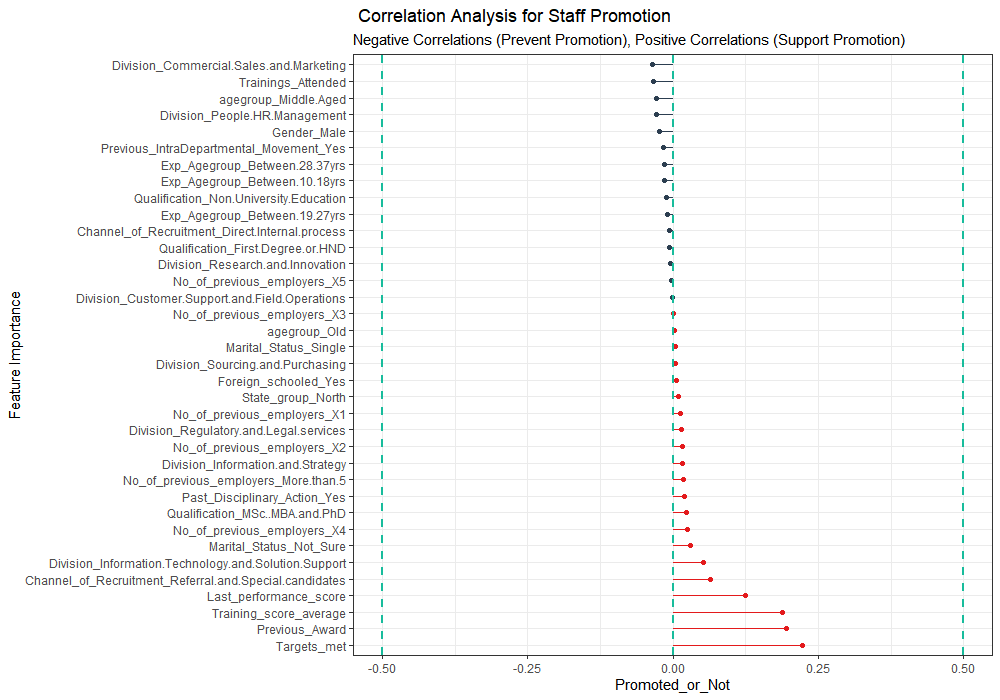The features with the red lines increase the likelihood of being promoted while the variables with blue lines decrease the likelihood of being promoted. From this correlation plot, we can see the features that contribute positively to staff promotion and those that prevent staff promotion.

# Conclusion

In this article, we applied machine learning techniques to examine factors that affect staff promotion based on the given data set. We started by splitting the dataset into training and test datasets. We implemented four machine learning algorithms namely: Random Forest, Logistic Regression, XGBoost and Support Vector Machine. The models were implemented using Caret Package in R. The performance of the trained models was evaluated on the test data set and evaluation metrics such as Accuracy and ROC curve were used. The results of the performance metrics showed that XGBoost performed better than other machine learning models. The LIME function was used to explain the important features of the XGBoost locally while we used correlation analysis to gain a globalized understanding of important features of the Xgboost model. For future work, we can tune the parameters of the Xgboost model for an improved accuracy rate.